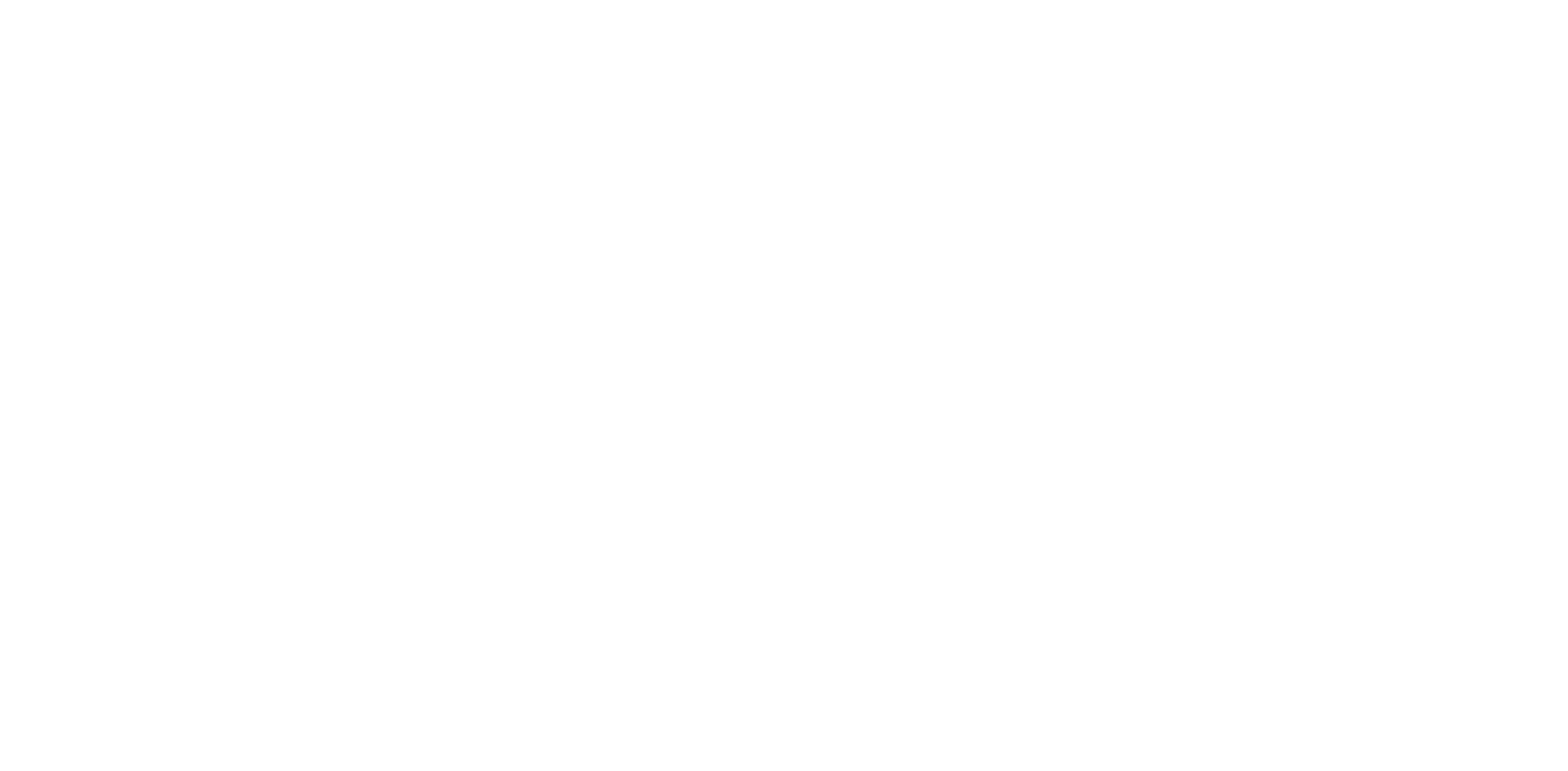AutoML.org

Freiburg-Hannover-Tübingen

# RoBO

RoBO – a Robust Bayesian Optimization framework written in python. The core of RoBO is a modular framework that allows to easily add and exchange components of Bayesian optimization such as different acquisition functions or regression models. It contains a variety of different regression models such as Gaussian processes, Random Forests or Bayesian neural networks and different acquisition function such as expected improvement, probability of improvement, lower confidence bound or information gain.
Besides standard Bayesian optimization methods, RoBO also includes the following state-of-the-art Bayesian optimization methods:

• Fabolas: Takes the training dataset size of an hyperparameter optimization benchmark as an additional input into account. This allows to evaluate single hyperparameter configuration on only subsets of data and extrapolate their performance on the full dataset in order to speed up the optimization procedure (see here) .
• Multi-Task Bayesian optimization: By providing an additional auxiliary task that is similar but cheaper to evaluate than the task of interest, MTBO can speed up the optimization procedure by evaluating configuration on the auxiliary task (see here).
• BOHamiANN: Uses Bayesian neural networks instead of Gaussian processes which are (because of the cubic complexity of GPs) more efficient with a large amount of data points. It uses stochastic gradient Hamiltonian Monte Carlo to sample from the posterior of the weights of the neural network.